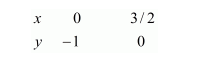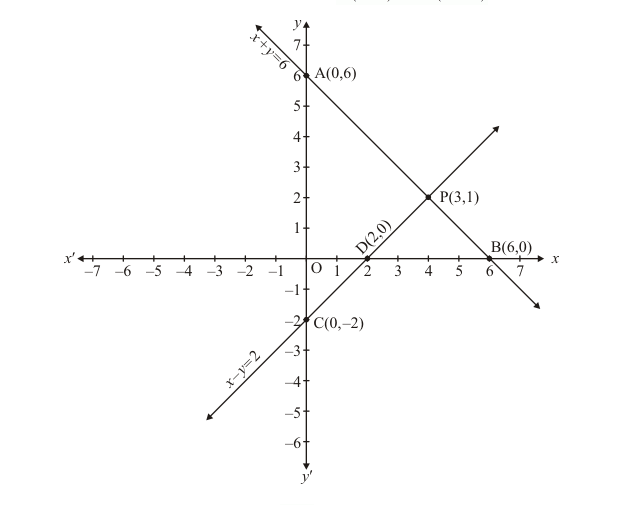# Solve the following systems of equations graphically:`
Question:

Solve the following systems of equations graphically:

$x+y=4$

$2 x-3 y=3$

Solution:

The given equations are

$x+y=4 \quad \ldots \ldots .(i)$

$2 x-3 y=3$ ..(ii)

Putting $x=0$ in equation $(i)$, we get:

$\Rightarrow 0+y=4$

$\Rightarrow y=4$

$\therefore x=0, \quad y=4$

Putting $y=0$ in equation $(i,$, we get

$\Rightarrow x+0=4$

$\Rightarrow x=4$

$\therefore x=4, \quad y=0$

Use the following table to draw the graph.Draw the graph by plotting the two points $A(4,0)$ and $B(4,0)$ from table.

Graph of the equation.... (ii):

$2 x-3 y=3$....(ii)

Putting $x=0$ in equation (ii) we get

$\Rightarrow 0-3 y=3$

$\Rightarrow y=-1$

$\therefore x=0, \quad y=-1$

Putting $y=0$ in equation $(i i)$, we get:

$\Rightarrow 2 x-0=3$

$\Rightarrow x=3 / 2$

$\therefore x=3 / 2, \quad y=0$

Use the following table to draw the graph.Draw the graph by plotting the two points $C(0,-1)$ and $D(3 / 2,0)$ from table.The two lines intersect at points $\mathrm{P}(3,1)$.

Hence $x=3, \quad y=1$ is the solution